# 【JS 逆向百例】DOM事件断点调试，某商盟登录逆向## 逆向目标

• 目标：某商盟登录
• 逆向参数：Query String Parameters：j_mcmm: 351faaef3ba8f4db2001ec621344dbbf

## DOM 简介

DOM 全称 Document Object Model，即文档对象模型，是 HTML 和 XML 文档的编程接口，定义了访问和操作 HTML 文档的标准方法。

<!DOCTYPE html>
<html>

<meta charset="utf-8">
<title>我的第一个HTML页面</title>

<body>
<h1>我的第一个标题</h1>
<p>我的第一个段落</p>
</body>

</html>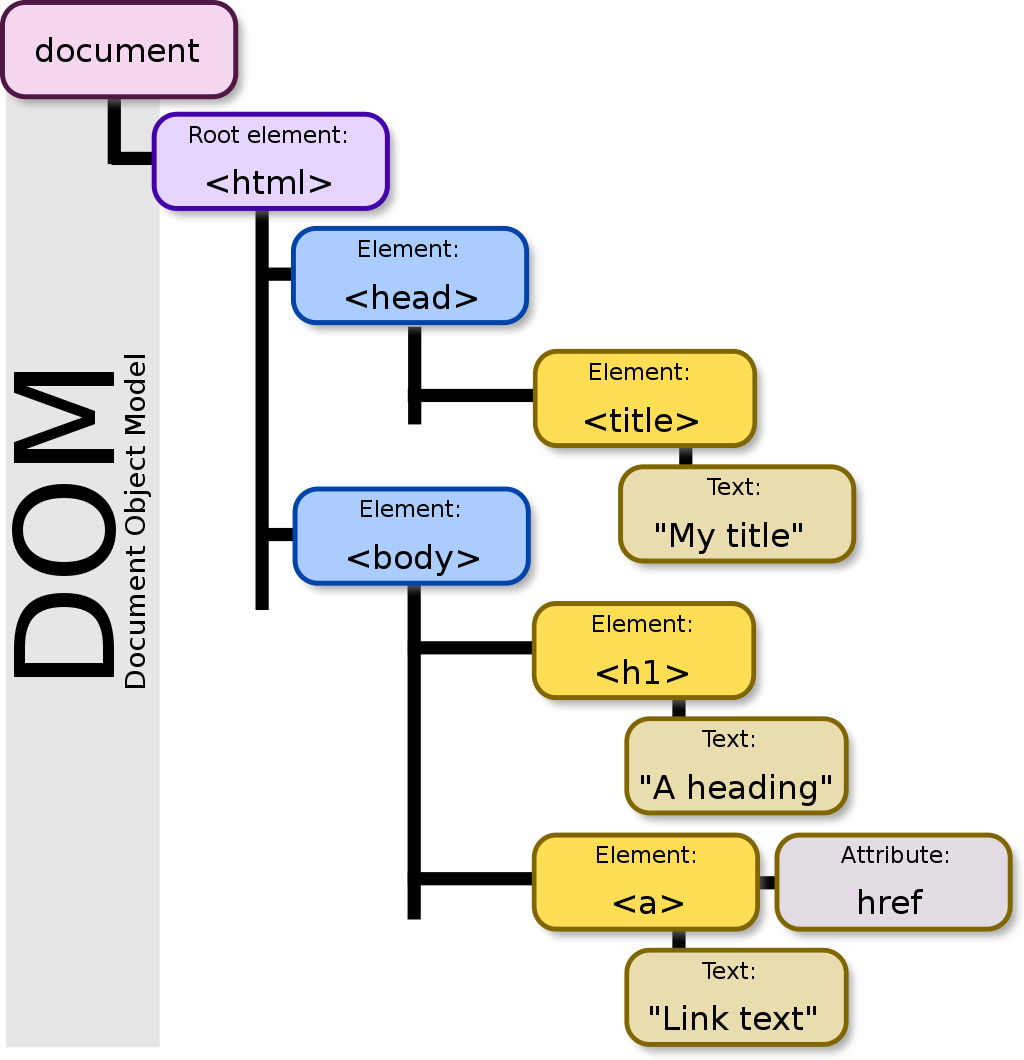## 逆向过程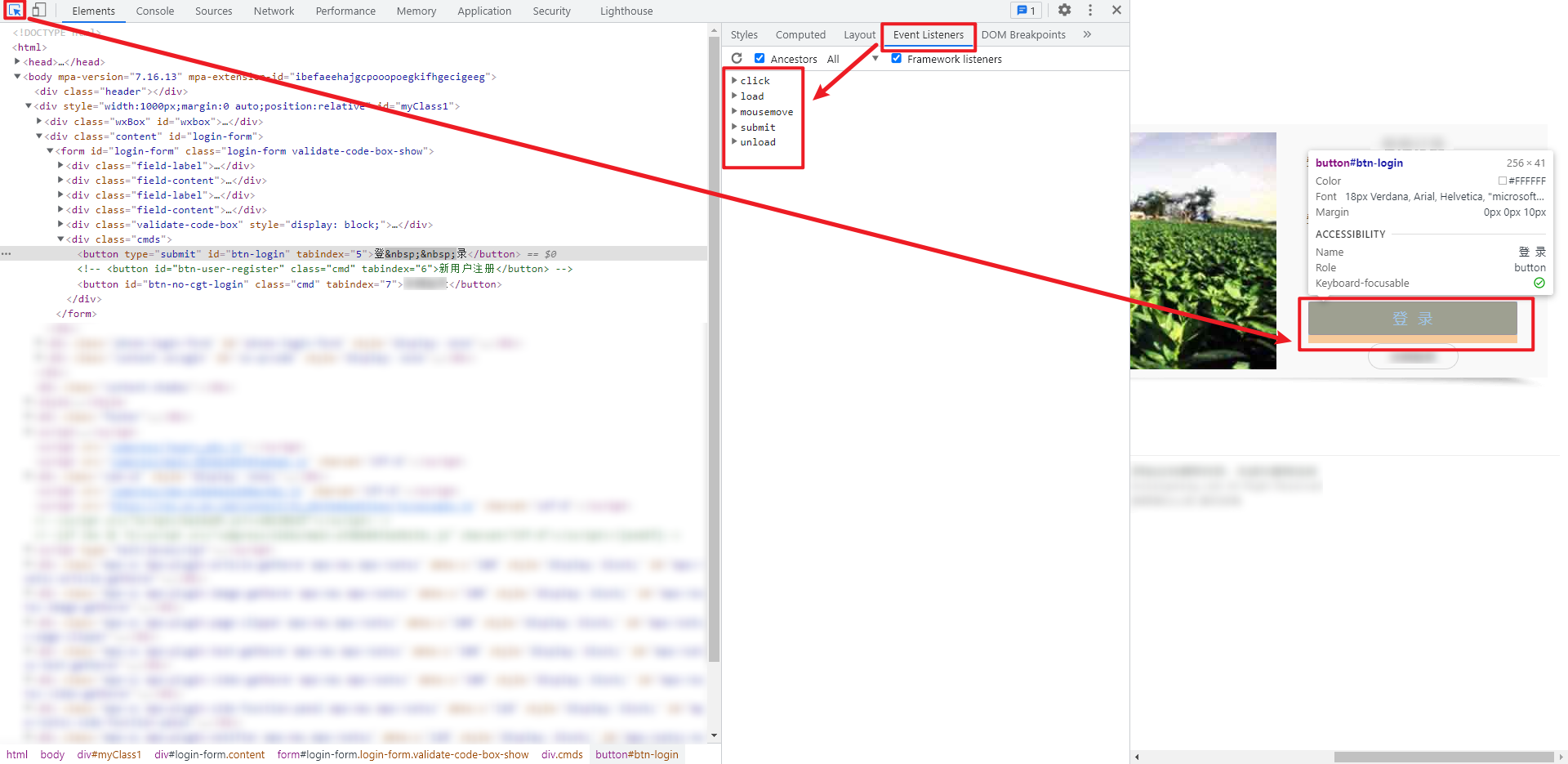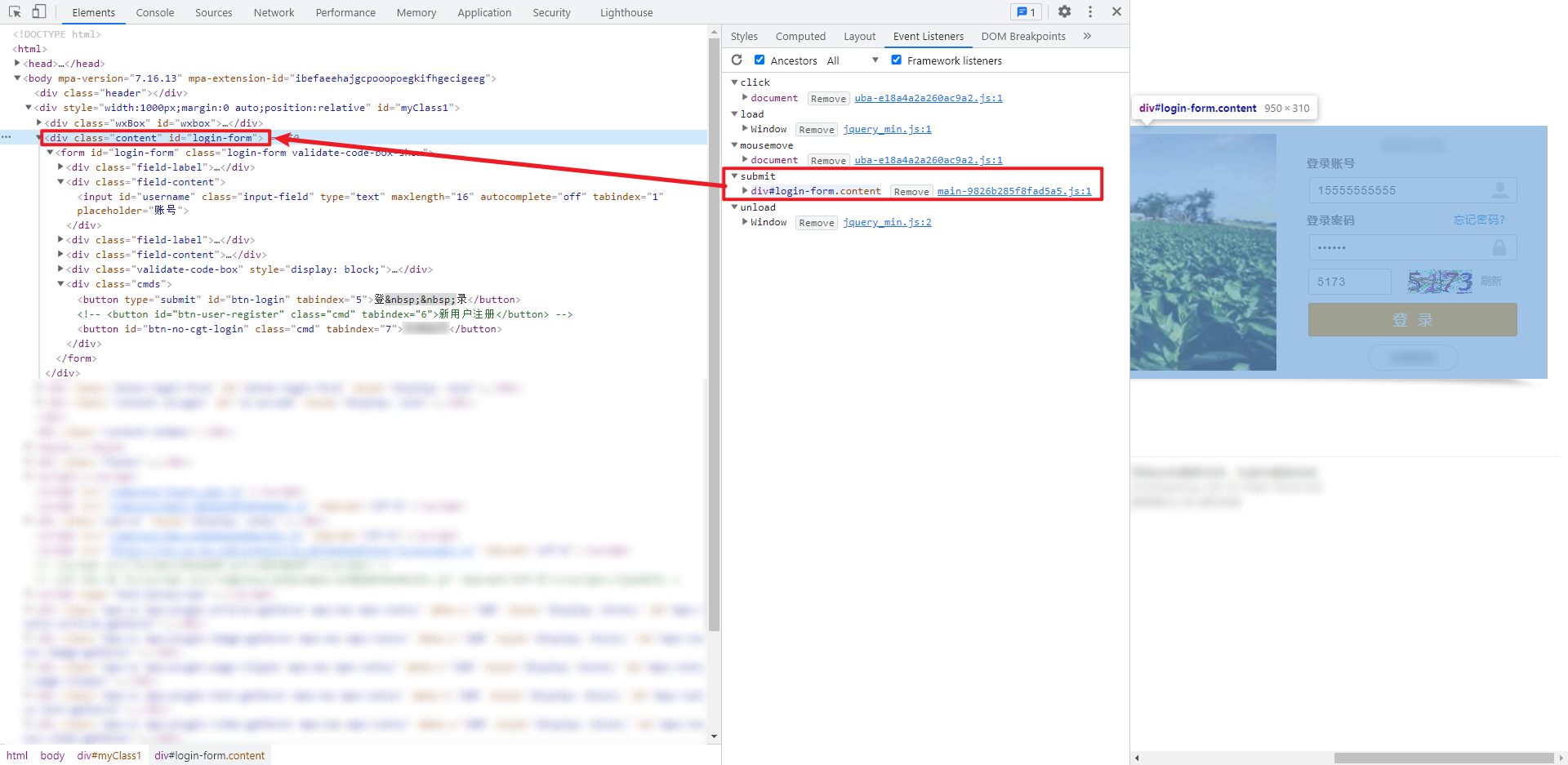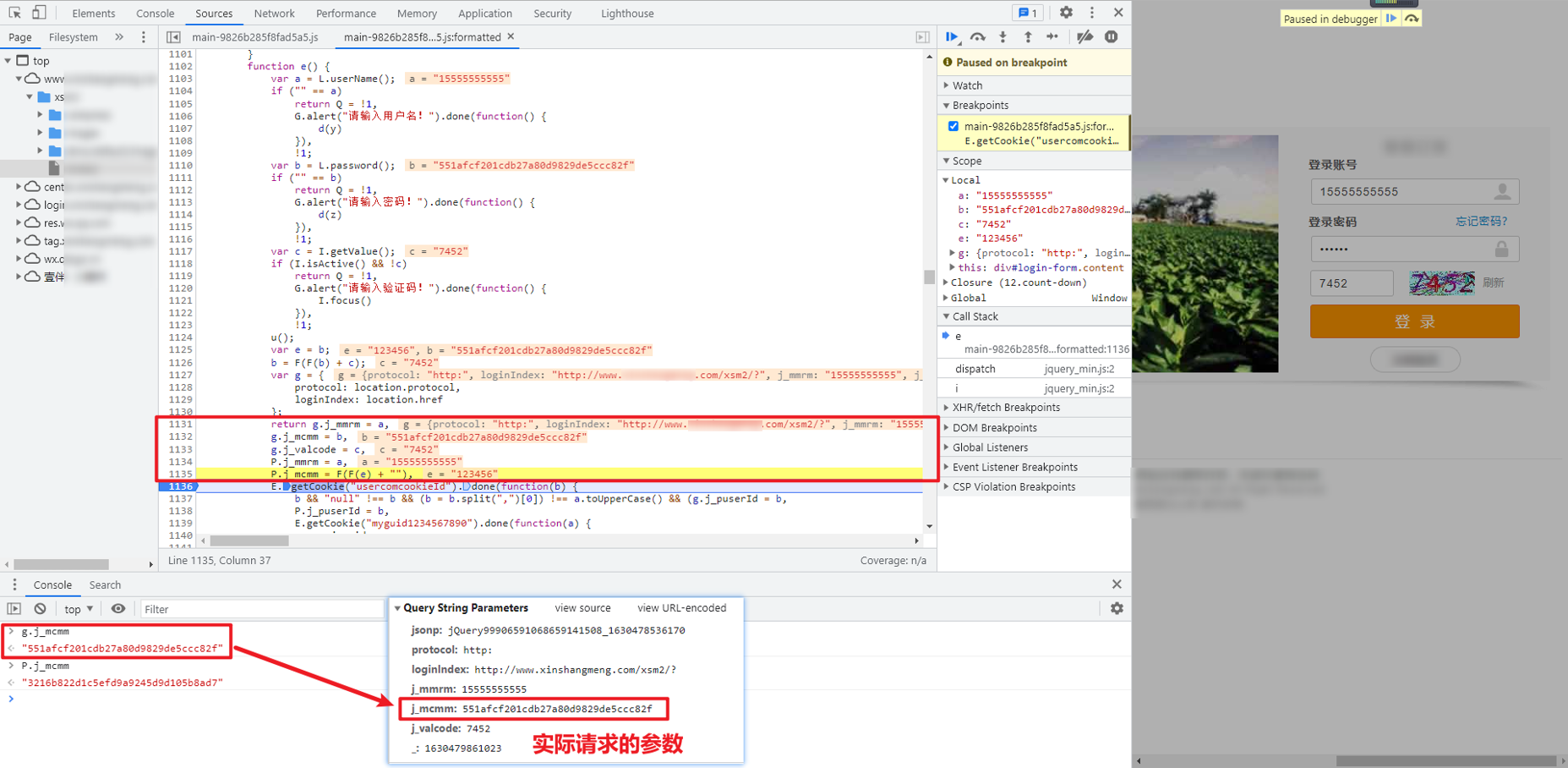g.j_mcmm = b 语句中，b 的值就是最终加密后的值，往上找，第 1125 和 1126 行 var e = b; b = F(F(b) + c);，把明文密码赋值给 b，c 为验证码，经过 F 这个函数的处理后得到加密值，继续跟进 F 函数：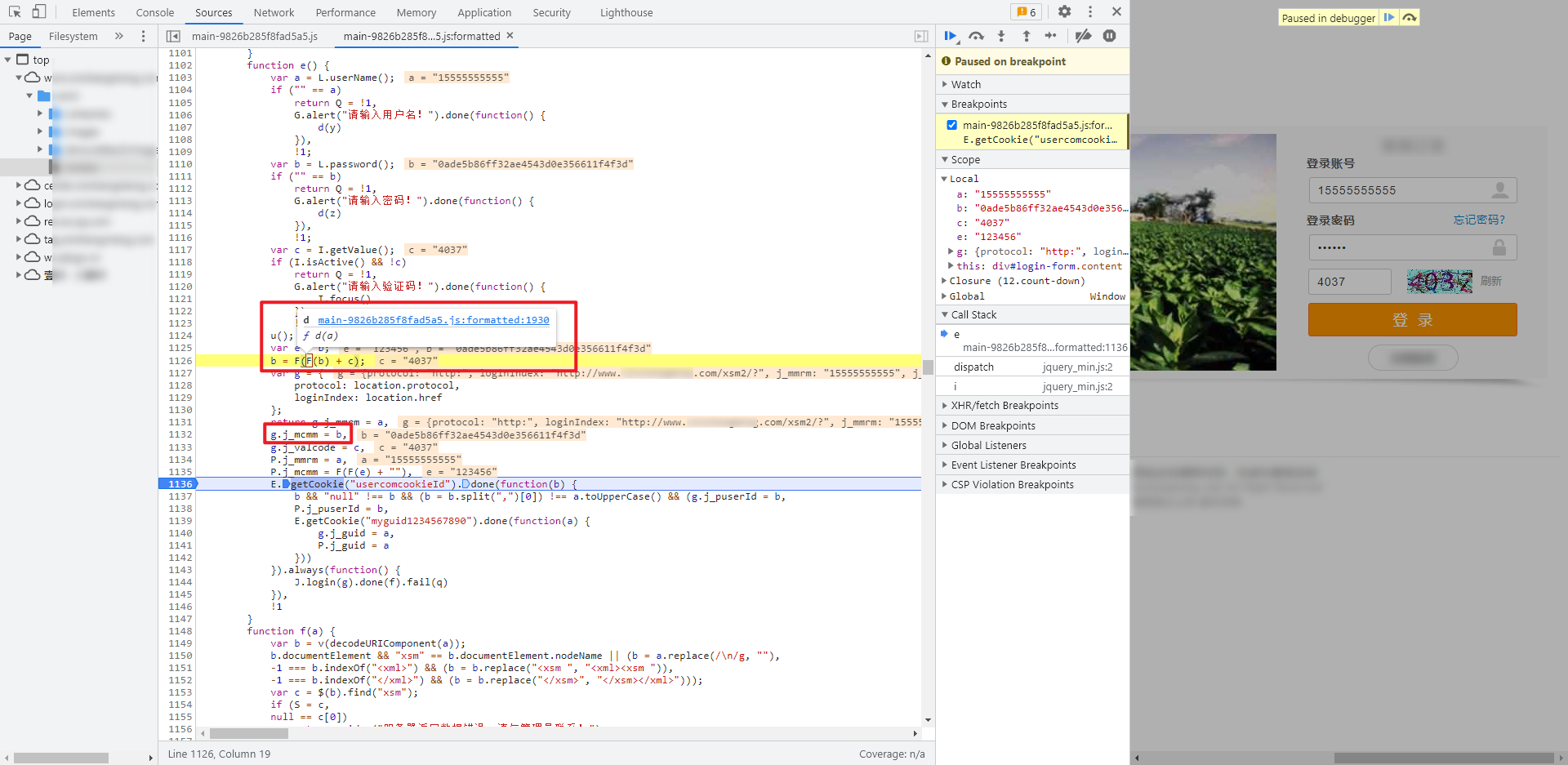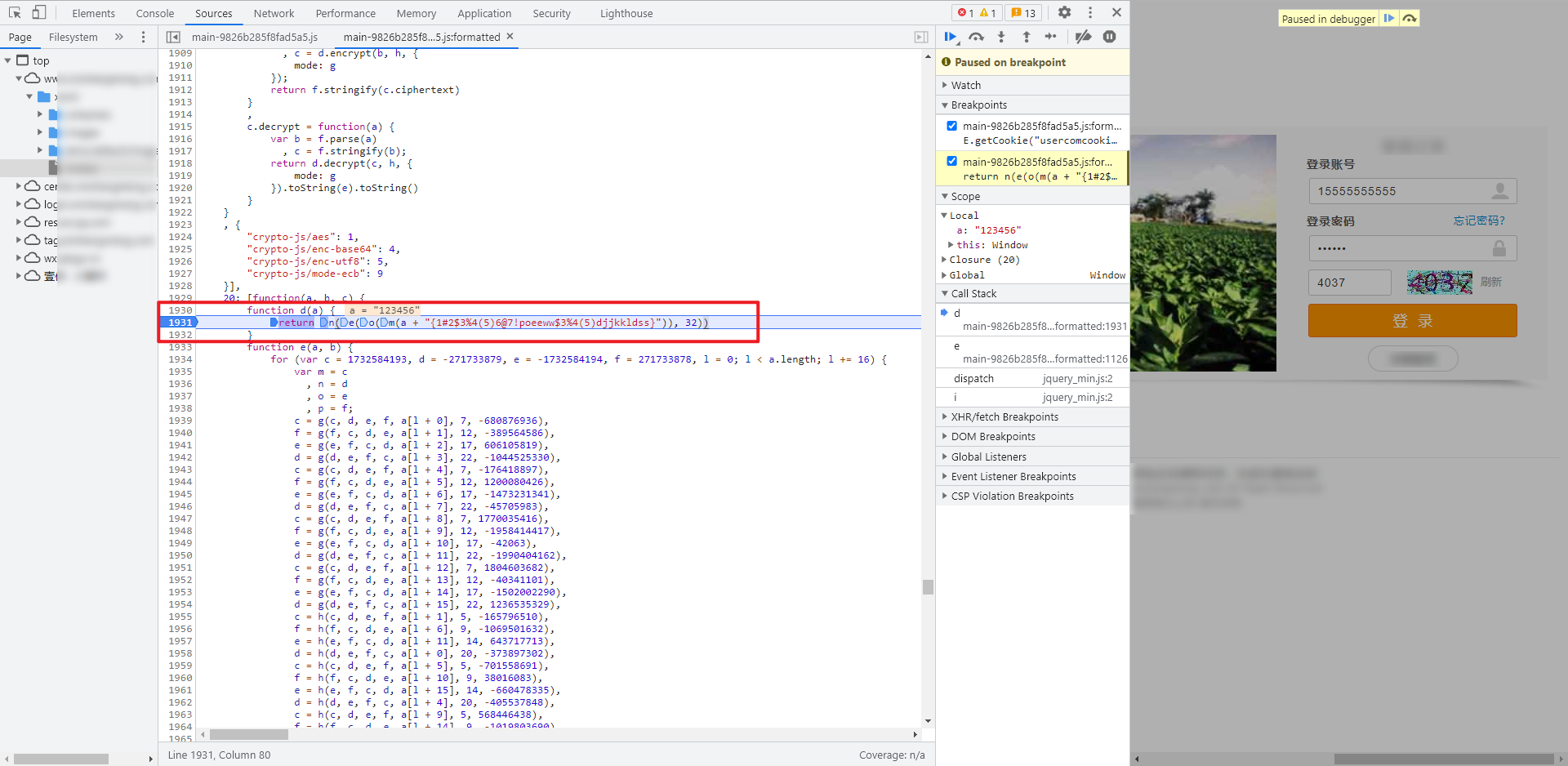function d(a) {
return n(e(o(m(a + "{1#2$3%4(5)6@7!poeeww$3%4(5)djjkkldss}")), 32))
}


## 完整代码

GitHub 关注 K 哥爬虫，持续分享爬虫相关代码！欢迎 star ！https://github.com/kgepachong/

### JavaScript 加密关键代码架构

function getEncryptedPassword(a, b, c) {
// a: 用户名, b: 密码, c: 验证码
function d(a) {
return n(e(o(m(a + "{1#2$3%4(5)6@7!poeeww$3%4(5)djjkkldss}")), 32))
}

function e(a, b) {}

function f(a, b, c, d, e, f) {}

function g(a, b, c, d, e, g, h) {}

function h(a, b, c, d, e, g, h) {}

function i(a, b, c, d, e, g, h) {}

function j(a, b, c, d, e, g, h) {}

function k(a, b)  {}

function l(a, b)  {}

function m(a)  {}

function n(a)  {}

function o(a)  {}

c.hex_md5 = d
b = d(d(b) + c);
return b
}

// 测试样例


### Python 登录关键代码

#!/usr/bin/env python3
# -*- coding: utf-8 -*-

import time
import random

import execjs
import requests
from PIL import Image

index_url = '脱敏处理，完整代码关注 GitHub：https://github.com/kgepachong/crawler'
ver_code_url = '脱敏处理，完整代码关注 GitHub：https://github.com/kgepachong/crawler'
'Referer': '脱敏处理，完整代码关注 GitHub：https://github.com/kgepachong/crawler',
'User-Agent': 'Mozilla/5.0 (Windows NT 10.0; Win64; x64) AppleWebKit/537.36 (KHTML, like Gecko) Chrome/91.0.4472.114 Safari/537.36'
}
session = requests.session()

def get_verification_code():
with open('code.png', 'wb') as f:
f.write(response.content)
image = Image.open('code.png')
image.show()
code = input('请输入验证码: ')
return code

with open('encrypt.js', 'r', encoding='utf-8') as f:

timestamp = str(round(time.time() * 1000))
jsonp = ''
for _ in range(20):
jsonp += str(random.randint(0, 9))
jsonp = 'jQuery' + jsonp + '_' + timestamp
params = {
'jsonp': jsonp,
'protocol': ' http:',
'j_valcode': code,
'_': timestamp
}
response.encoding = 'utf-8'
print(response.text)

def main():